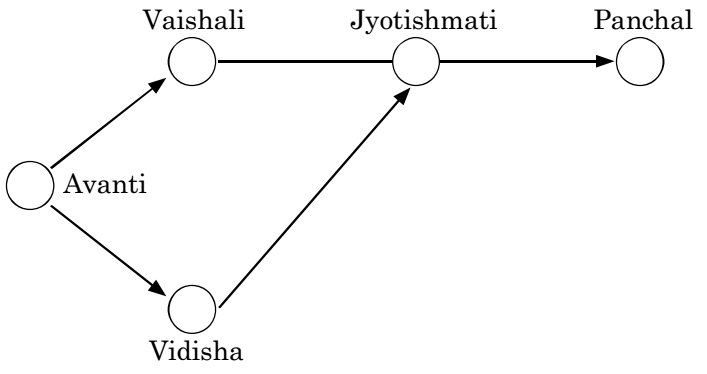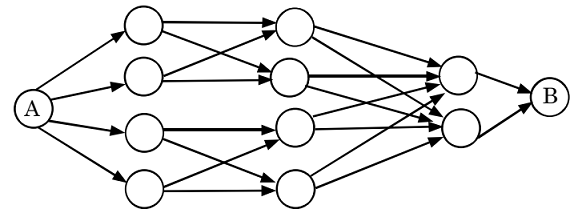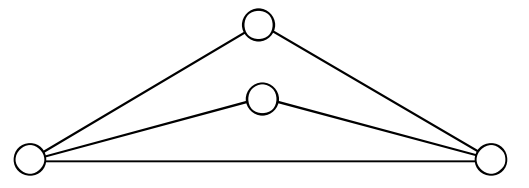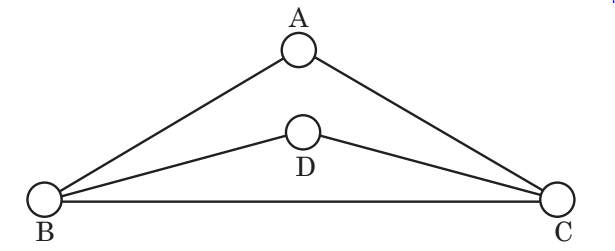## Routes and Networks

#### Routes and Networks

Direction: The following sketch shows the pipelines carrying material from one location to another. Each location has a demand for material. The demand at Vaishali is 400, at Jyotishmati is 400, at Panchal is 700, and at Vidisha is 200. Each arrow indicates the direction of material flow through the pipeline. The flow from Vaishali to Jyotishmati is 300. The quantity of material flow is such that the demands at all these locations are exactly met. The capacity of each pipeline is 1,000.1. The quantity moved from Avanti to Vidisha is
1. 200
2. 800
3. 700
4. 1,000

1. As per the given above figure , we can see that
Since flow from Vaishali to Jyotishmati is 300 where as demand is 400 so the deficient 100 would be met by flow from Vidisha.
Again the demand of 700 in Panchal is again to be met by flow from Jyotishmati which can get it from Vidisha.

##### Correct Option: D

As per the given above figure , we can see that
Since flow from Vaishali to Jyotishmati is 300 where as demand is 400 so the deficient 100 would be met by flow from Vidisha.
Again the demand of 700 in Panchal is again to be met by flow from Jyotishmati which can get it from Vidisha.
Thus, the quantity moved from Avanti to Vidisha is 200 + 100 + 700 = 1000

1. What is the free capacity available in the Avanti-Vidisha pipeline?
1. 300
2. 200
3. 100
4. 0

1. On the basis of above given route network diagram , we can see that
Free capacity in Avanti-Vidisha is zero.
Explanation is similar as in previous answer.

##### Correct Option: D

On the basis of above given route network diagram , we can see that
Free capacity in Avanti-Vidisha is zero.
Explanation is similar as in previous answer.

1. The free capacity available at the Avanti-Vaishali pipeline is
1. 0
2. 100
3. 200
4. 300

1. From above given route diagram , we can see that
Free capacity at Avanti-Vaishali pipeline is 300, since capacity of each pipeline is 1000 and demand at Vidisha is 400 and 300 flows to Jyotishmati.

##### Correct Option: D

From above given route diagram , we can see that
Free capacity at Avanti-Vaishali pipeline is 300, since capacity of each pipeline is 1000 and demand at Vidisha is 400 and 300 flows to Jyotishmati.
Thus, free capacity = {1000 – (400 + 300)} = 300

1. What is the total number of ways to reach A to B in the network given?1. 12
2. 16
3. 20
4. 22

1. As per the given above figure , we can see that
There are four ways to go from A to the first level of nodes. Each of these 4 nodes in turn leads into two more ways to go to the second level nodes.
Each of the second level nodes leads into two more ways to go to the third level nodes.

##### Correct Option: B

As per the given above figure , we can see that
There are four ways to go from A to the first level of nodes. Each of these 4 nodes in turn leads into two more ways to go to the second level nodes.
Each of the second level nodes leads into two more ways to go to the third level nodes.
And from here we have only one way each to go to B.
Hence by fundamental principal of counting, total number of ways = 4 x 2 x 2 x 1 = 16 ways.

1. 4 cities are connected by a road network as shown in the figure. In how many ways can you start from any city and come back to it without travelling on the same road more than once?1. 8
2. 12
3. 16
4. 20

1. According to question ,
It can be seen that every city is connected to all the other cities (i.e. 3 other cities).
Step 1: Let starting point is A, there are 3 ways in which we could proceed, viz. AB, AD or AC.
Step 2: Once we are at any of these cities (B, D or C), each one of them is connected to 3 other cities. But since we cannot go back to A the originating city, there are only 2 ways in which we could proceed from here.

##### Correct Option: B

According to question ,
It can be seen that every city is connected to all the other cities (i.e. 3 other cities).
Step 1: Let starting point is A, there are 3 ways in which we could proceed, viz. AB, AD or AC.
Step 2: Once we are at any of these cities (B, D or C), each one of them is connected to 3 other cities. But since we cannot go back to A the originating city, there are only 2 ways in which we could proceed from here.
Step 3: let us assume that we are at B, we can only go to D or C by taking BD or BC respectively. From this point we a choice of either directly going back to A (thus skipping 4th city or go to 4th city and come back to A. )
Step 4:- Now if we are at D, we can either take DA or DCA. So there are 2 more ways to go from here.
So , total number of ways = 3 x 2 x 2 = 12 ways.